# Noise Figure

Click here to go to our page on low noise amplifiers (LNAs)

Click here to go to our page on using source pull to measure noise parameters

Keysight has the world's best app notes on noise figure, here's a link to a good one! Also, the IEEE Long Island section of IEEE MTT-S has some good lecture notes on noise that are worth checking out.

The weakest signal a receiver can discriminate is a function of how much thermal noise the receiver adds to the signal. The signal-to noise ratio is the most convenient way of quantifying this effect. Harald Friis is the first engineer to develop the theory of noise figure, and for this he is in the Microwave Hall of Fame!

### Signal to noise ratio (S/N)

This is a measure of how far a signal "pokes through" the noise floor. By looking at a spectrum analyzer display, you'll understand what this means. More to come!

### Noise factor versus noise figure

Noise factor is a measure of how the the signal to noise ratio is degraded by a device:

F=noise factor=(Sin/Nin)/(Sout/Nout)

Where Sin is the signal level at the input

Nin is the noise level at the input,

Sout is the signal level at the output

and Nout is the noise level at the output.

The noise factor of a device is specified with noise from a noise source at room temperature (Nin=KT), where K is Boltzman's constant and T is approximately room temperature in Kelvin; KT is somewhere around -174 dBm/Hz. Depending on where devices are positioned in an amplification chain, the individual noise factors will have different effects on the overall noise, according to Friis (see below). Thanks for clarifying this, Andreas!

Signal to noise ratio always worsens from input to output due to entropy or one of those other depressing laws of the universe; as the S/N ratio at output is less than S/N ratio at input, noise factor is always greater than unity.

Noise figure is the noise factor, expressed in decibels:

NF (decibels)=noise figure =10*log(F)

Noise figure is more often used in microwave engineering, but noise calculations use the noise factor, as we will soon see.

Starting May 2009, our discussion of noise temperature has been moved to a separate page.

### Noise figure of passive devices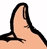Time for another Microwaves101 rule of thumb!

Linear passive devices have noise figure equal to their loss. Expressed in dB, the NF is equal to -S21(dB). Something with one dB loss has one dB noise figure.

### Cascading noise figure in a system

See our page on cascade analysis.

The noise factor contributions of each stage in a chain follow this equation: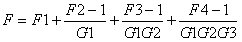This is known as the Friis equation, after Harald Friis. Let's look at an example of how this math works. Below is a receiver chain schematic that we whipped up using the Microwaves101 download for schematic symbols that you can grab from our download area. For an explanation on the filter schematic symbols, go here. Our proposed receiver chain has a preselector filter in front of the LNA to limit the amount of stray signals that might come in and saturate it. Following the LNA is a image rejection filter, to prevent image noise foldover (we'll explain that some other time, we promise!) Then the mixer and IF amp round out the chain.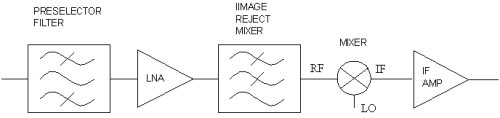Now let's look at how to apply the Friis equation. We made a very simple spreadsheet that calculates the noise figure as it cascades down the chain. We made up some typical values for the gain and noise figure of each piece. The LNA usually has lower noise figure than the IF amp.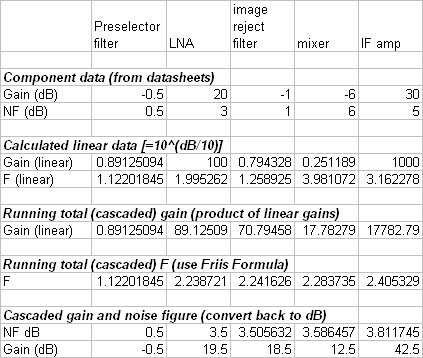The gain calculation of the chain is trivial, but let's look at the the Friis equation as it calculates noise factor through the chain: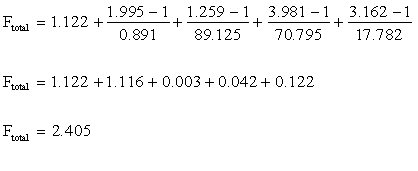Expressing the noise factor in decibels, we arrive at the noise figure of 3.81 that our simple spreadsheet calculated. By the way, don't spend any time thinking about gain and noise figure beyond one or two decimal digits, in practice, you'll never be able to measure the difference between 3.812 and 3.8 dB.

The relative noise contribution of the five stages shows that after the LNA, not much else is added to the noise factor. After the first two stages (F=1.122 and F=1.116), the noise factor at this point is 93% of the entire chain. Whenever your chain has a lot of gain up front, the denominator of subsequent stages in the Friis equation tends to wipe out the noise factor of the numerator.Time for another Microwaves101 rule of thumb!

If you have 20 dB gain in your LNA or receiver, the noise figure contribution of the subsequent stage will be small (unless the noise figure of the next stage is horrendous!) In our example above, the preselector filter/LNA had 19.5 dB gain and 3.5 dB noise figure. The combined noise figure of the filter, mixer and IF amp which follow is 12 dB, yet it only degrades the noise figure of the entire lineup by 0.3 dB!

More to come soon! We'll cover the topic of noise figure measurement another day.

Author : Unknown Editor